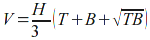SEARCH HOMEMath Central Quandaries & QueriesQuestion from A. Ahamed: what is the formula to find out volume of a pyramid with individual length and breadth? For example bottom size is 6.5m x 6.5m, top size 1.5m x 4.2m and height 0.8m. please send me the formula.Hi there.

The volume of a pyramid is the area of the base times the height, then divided by 3. What you are describing is a pyramid with the top missing, so that's just a pyramid minus a pyramid.

We call it a frustum.

Since you know the areas of the parallel top (T) and bottom (B) and the height between them (H), you can use the formulaHope this helps,
Stephen La RocqueMath Central is supported by the University of Regina and The Pacific Institute for the Mathematical Sciences.